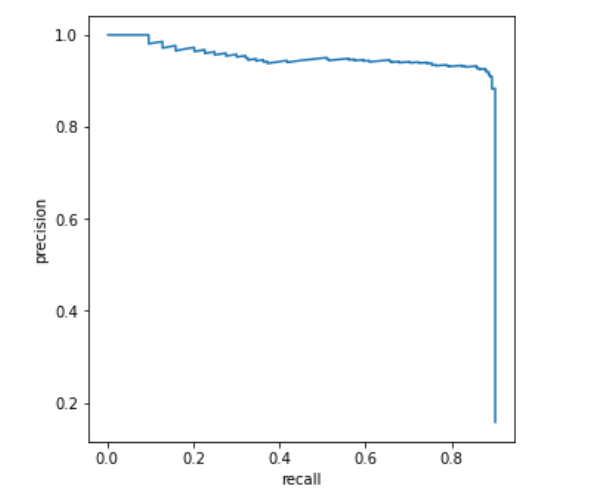Fenrier Lab

# 对目标检测模型 PR 曲线的原理分析和实现

PR曲线其实就是以准确率(Precise)为横轴，召回率(Recall)为纵轴的曲线，定义很简单，但是我们立即会产生一个疑问，那就是对于一个确定的模型，怎么准确率咋还能变化呢？所以要理解 PR 曲线，首先需要知道准确率和召回率是怎么变化的。

|gt_id|pred_id|iou|confidence| |–|–|–|–|–|–|

pred_boxes: Boxes = ...
gt_boxes: Boxes = ...
iou_matrix: torch.Tensor = pairewise_iou(pred_boxes, gt_boxes)
ious, gt_ids = iou_matrix(dim=0)


df_i = pd.DataFrame({
"gt_id": [f"{image_id}_{i}" for i in gt_ids.cpu().numpy().tolist()],
"predict_id": [f"{image_id}_{i}" for i in range(len(gt_ids))],
"iou": ious.cpu().numpy().tolist(),
"confidence": output["instances"].scores.cpu().numpy().tolist()
}


df=pd.concat((df, df_i), axis=0)


index gt_id predict_id iou confidence
0 0006990_1 0006990_0 0.922708 0.999956
1 0006990_5 0006990_1 0.880718 0.999952
2 0006990_14 0006990_2 0.986290 0.999944
3 0006990_26 0006990_3 0.942946 0.999930
4 0006990_24 0006990_4 0.926946 0.999927

df["TP"] = df["confidence"] > 0.5


max_iou=df[["gt_id", "iou"]].groupby("gt_id").max()
best_match_df=df[["gt_id", "iou", "predict_id"]].merge(max_iou, how="inner", on = ["gt_id", "iou"])
best_match_df=best_match_df.drop(columns=["iou"])
best_match_df["best_match"]=pd.Series([True for _ in range(len(best_match_df))])


index gt_id predict_id best_match
0 0006990_1 0006990_0 True
1 0006990_5 0006990_1 True
2 0006990_14 0006990_2 True
3 0006990_26 0006990_3 True
4 0006990_24 0006990_4 True

df = df.merge(best_match_df, how="outer", on=["gt_id", "predict_id"]).fillna(False)


df["TP"] = df["TP"] & df["best_match"]


df = df.sort_values(by="confidence", ascending=False)

N = len(pd.unique(df["gt_id"]))

M = pd.Series([i + 1 for i in range(len(df))])

P = pd.Series([tp[0:i+1].sum() for i in range(len(df))])

precise = P / M
recall = P / N